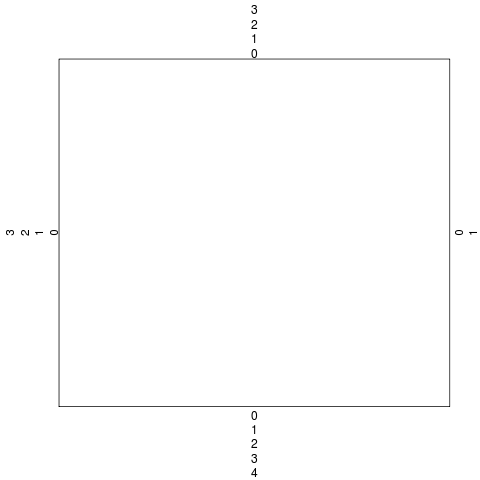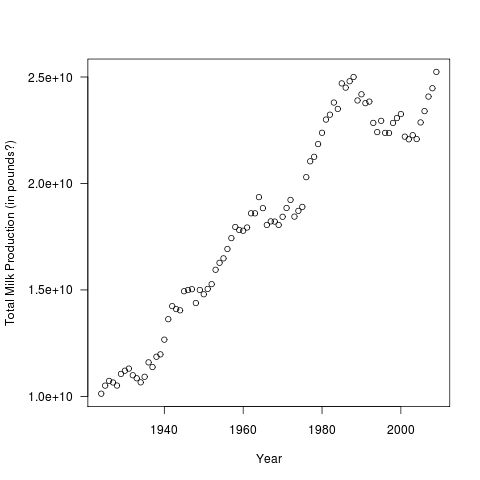[This article was first published on The Pretty Graph Blog » R, and kindly contributed to R-bloggers]. (You can report issue about the content on this page here)
Want to share your content on R-bloggers? click here if you have a blog, or here if you don't.

It is fairly straightforward to set the margins of a graph in R by calling the par() function with the mar (for margin!) argument. For example,

par(mar=c(5.1,4.1,4.1,2.1)

sets the bottom, left, top and right margins respectively of the plot region in number of lines of text.

Another way is by specifying the margins in inches using the mai argument:

par(mai=c(1.02,0.82,0.82,0.42))

The numbers used above are the default margin settings in R. You can verify this by firing up the R prompt and typing par(“mar”) or par(“mai”). You should get back a vector with the above values. The bottom, left and top margins are the largest because that’s where annotations and titles are most likely to be placed.

Since we can specify margins both in terms of lines of text and inches, let’s find out how high one line of text is by default:

par(“mai”)/par(“mar”)
 0.2 0.2 0.2 0.2

0.2 inches!

There are ways to change this line height but that’s a useful number to keep in mind.

The default size of the figure region is approximately 7 inches wide by 7 inches high. You can verify this by typing par(“fin”) at the R prompt. So, by default the figure is 35 lines high and wide. One way to verify this is by trying to run the following code:

par(mar=c(35,35,0,0))
plot(1:10)

What happens? We get an error saying “figure margins too large”. That was bound to happen because we used up all of the figure region in margins and left no space for the plot to be drawn! You are probably never going to set such large margins, but in my experience errors like that occur when I’m working with multiple plot layouts (using the mfrow argument – I might write a post about that some time).

Margin lines are numbered starting from 0. We already know the number of margin lines from par(“mar”) but let’s make a graph to illustrate this point and see how the margin lines are numbered:

plot(1:10,ann=FALSE,type=”n”,xaxt=”n”,yaxt=”n”)
for(j in 1:4) for(i in 0:10) mtext(as.character(i),side=j,line=i)In the above example, we used the mtext() function (which as the name suggests places text in the margins) to label the margin lines.

The mgp argument of the par() function is a vector of 3 values which specify the margin line for the axis title, axis labels and axis line. The default value of mgp is c(3,1,0), which means that the axis title is drawn in the fourth line of the margin starting from the plot region, the axis labels are drawn in the second line and the axis line itself is the first line.

Sometimes the axis labels may be very long and overlap with the axis title (for example, large numbers in scientific notation on the y axis). To overcome this we can use par() to first increase the left margin and then use mgp to set the axis title line. Note that using mgp applies the same set of margin values to axes on all four sides. Alternatively, we can suppress the drawing of the default axis label and use mtext() function specifying the line argument to a value higher than default. Let’s look at an interesting example to try this out.

Recently, there was a blog post showing some interesting data about the milk production powers of Wisconsin’s super-efficient cows. So, let’s pick up that data and plot the total milk production for the last few decades.

#This bit of code is to remove the commas in numerical fields in the original dataset. I don’t know of any automatic ways to do this in R.
for (i in 1:ncol(cows)){
if(length(grep(“,”,cows[[i]]))>0)
cows[[i]] <- as.numeric(gsub(",", "", cows[[i]]))
}

plot(cows\$Total.milk.production~cows\$Year,las=1,xlab=”Year”, ylab=”Total Milk Production (in pounds?)”)Now, you may argue that showing cow milk production in scientific (E) notation is a bit too nerdy, but I think it’s a good enough example here. The Y axis title overlaps the axis labels, making the graph hard to read and a bit ugly. So, let’s fix it by increasing the left margin using mar and placing the axis title in a higher margin line using mgp:

par(mar=c(5,6,4,2)+0.1,mgp=c(5,1,0))
plot(cows\$Total.milk.production~cows\$Year,las=1,xlab=”Year”, ylab=”Total Milk Production (in pounds?)”)That looks better, but did you notice we knocked out the X axis title? That happened because as I wrote earlier, mgp applies to both the axes. So we asked par to place the axes titles in line number 5. Since line numbering starts at 0, that’s the sixth line in the margin. But we only left 5 lines worth of margin space on the bottom X axis. So the X axis title did not fit within the figure.

To get around this problem, there are at least three solutions. Let’s first look at the hardest one.

par(mar=c(5,6,4,2)+0.1)
plot(cows\$Total.milk.production~cows\$Year,las=1,xlab=”Year”, ylab=””)
mtext(“Total Milk Production (in pounds?)”,side=2,line=5)Voila! So we dropped the mgp argument, set the left margin wide, suppressed the default Y axis label and then used mtext to place the title in line 5. Thus, we used the defaults for the X axis title and used a custom function call for the Y axis title.

What are the easy solutions then? Just use lattice or ggplot2 – they will take care of the margins automatically almost in all cases without you having to worry about it.

If you are wondering why I am wrestling with these base graphics settings, there is a good reason. I’m building a web-based graphing application using R, so I need to automatically and quickly create good looking graphs for a variety of use cases. In my experience, the base graphics functions are faster than using lattice and ggplot2 simply because loading the packages takes a few too many seconds. In building the code for Pretty Graph to handle all sorts of user input data and help people visualise them as different types of graphs, I am having to hack around the base R code to make it produce good graphs consistently. I think that one can make very good graphs using the basic functions if one spends some time learning the different parameters. This is the first in a series of blog posts where I talk about my experience in building R graphs and some interesting quirks of R graphics functions. I hope it will be a good learning experience for me.To leave a comment for the author, please follow the link and comment on their blog: The Pretty Graph Blog » R.

R-bloggers.com offers daily e-mail updates about R news and tutorials about learning R and many other topics. Click here if you're looking to post or find an R/data-science job.
Want to share your content on R-bloggers? click here if you have a blog, or here if you don't.

# Never miss an update! Subscribe to R-bloggers to receive e-mails with the latest R posts.(You will not see this message again.)

Click here to close (This popup will not appear again)